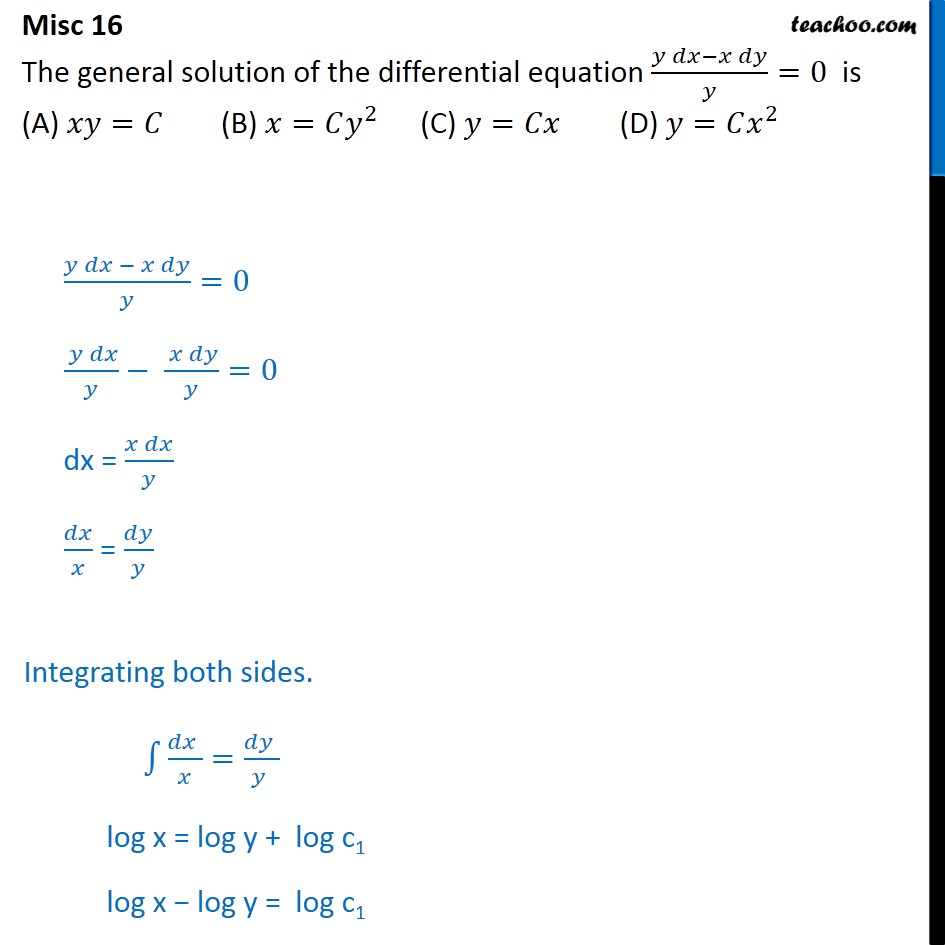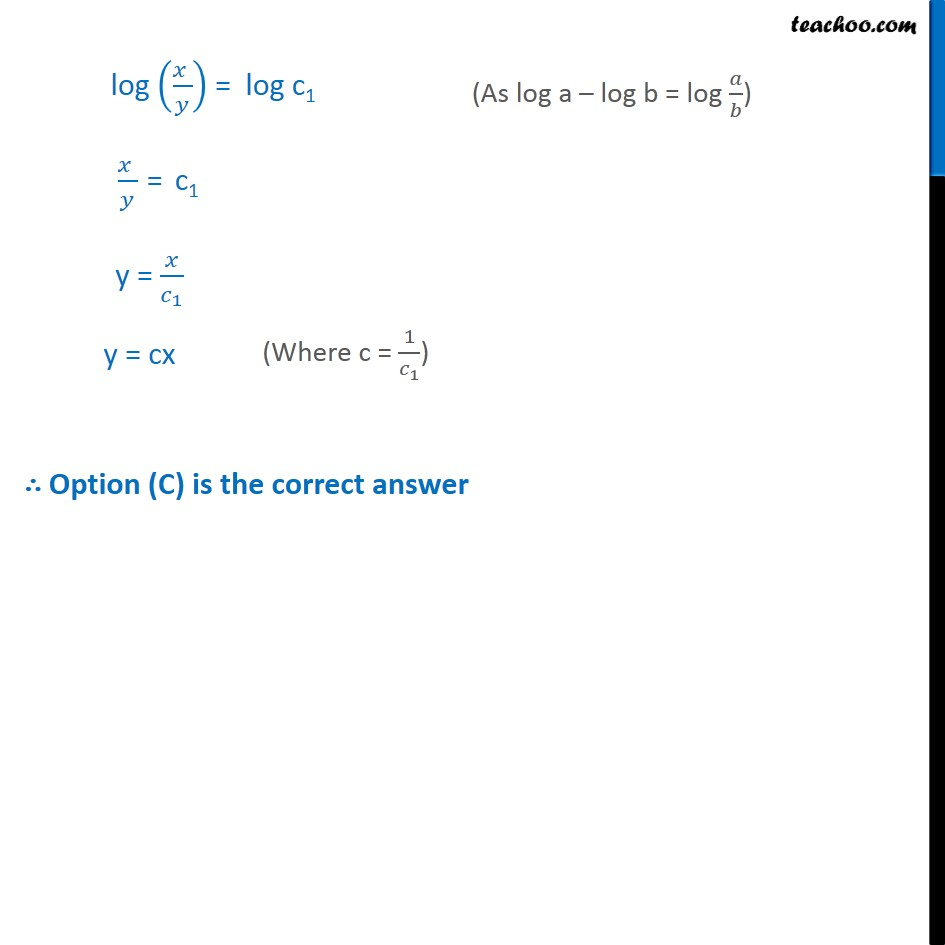Miscellaneous

Chapter 9 Class 12 Differential Equations
Serial order wiseLearn in your speed, with individual attention - Teachoo Maths 1-on-1 Class

### Transcript

Misc 16 The general solution of the differential equation (𝑦 𝑑𝑥−𝑥 𝑑𝑦)/𝑦=0 is (A) 𝑥𝑦=𝐶 (B) 𝑥=𝐶𝑦^2 (C) 𝑦=𝐶𝑥 (D) 𝑦=𝐶𝑥^2 (𝑦 𝑑𝑥 − 𝑥 𝑑𝑦)/𝑦=0 ( 𝑦 𝑑𝑥)/𝑦− ( 𝑥 𝑑𝑦)/𝑦=0 dx = (𝑥 𝑑𝑥)/𝑦 𝑑𝑥/𝑥 = 𝑑𝑦/𝑦 Integrating both sides. ∫1▒〖(𝑑𝑥 )/𝑥=(𝑑𝑦 )/(𝑦 )〗 log x = log y + log c1 log x − log y = log c1 log ((𝑥 )/𝑦) = log c1 (𝑥 )/𝑦 = c1 y = 𝑥/𝑐_1 y = cx ∴ Option (C) is the correct answer